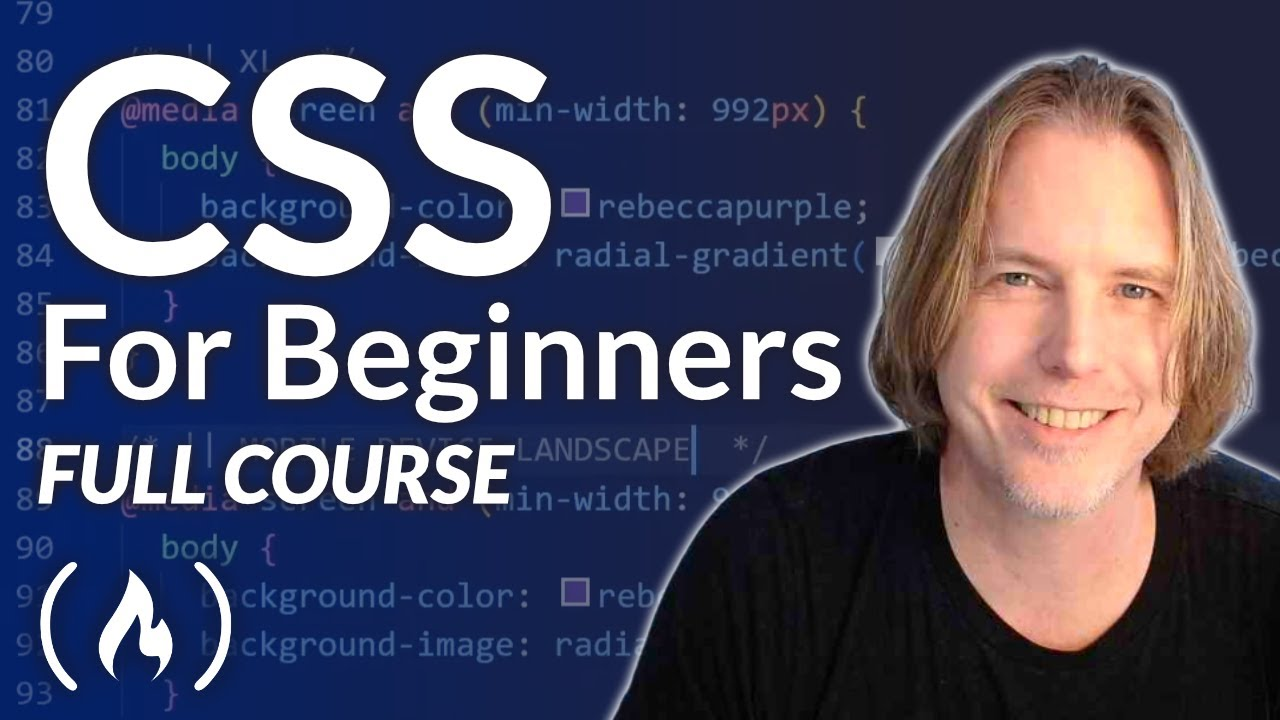# CSS Tutorial – Full Course for BeginnersIn this in-depth course, you will learn about all the key features of CSS. This is the most comprehensive CSS course we've published to date. So if you want to become an expert in Cascading Style Sheets, this is the course for you.

In this in-depth course, you will learn about all the key features of CSS. This is the most comprehensive CSS course we’ve published to date. So if you want to become an expert in Cascading Style Sheets, this is the course for you.

⭐️ Course Contents ⭐️
(0:00:00) Intro
(0:01:08) Chapter 1: Start Here
(0:14:50) Chapter 2: Selectors
(0:34:41) Chapter 3: Colors
(0:51:13) Chapter 4: Units & Sizes
(1:11:56) Chapter 5: Box Model
(1:37:08) Chapter 6: Typography
(2:16:37) Chapter 8: List Styles
(2:32:31) Chapter 9: Mini Project
(2:45:04) Chapter 10: Display
(3:00:21) Chapter 11: Floats
(3:12:46) Chapter 12: Columns
(3:34:30) Chapter 13: Position
(3:57:53) Chapter 14: Flexbox
(4:21:39) Chapter 15: Grid Layout
(4:46:33) Chapter 16: Images
(5:32:40) Chapter 17: Media Queries
(5:58:59) Chapter 18: Card Project
(6:33:21) Chapter 19: Pseudo
(6:52:56) Chapter 20: Variables
(7:20:28) Chapter 21: Functions
(7:50:05) Chapter 22: Animations
(8:37:33) Chapter 23: Organization
(8:57:23) Chapter 24: Final Project